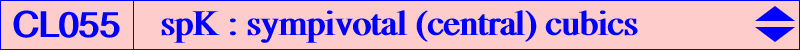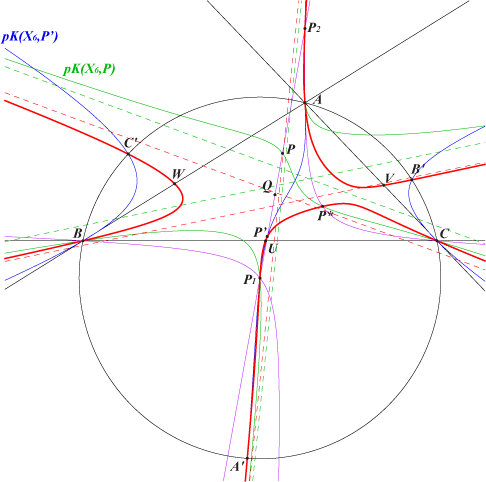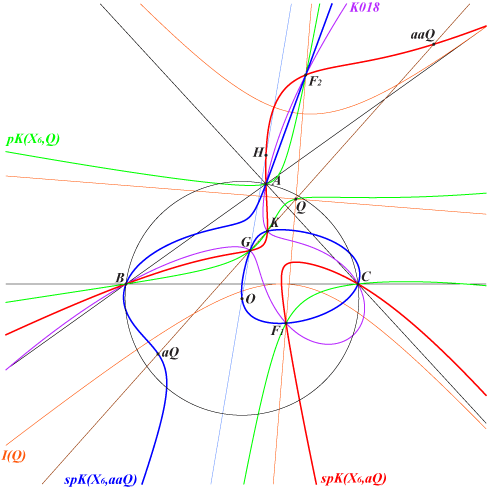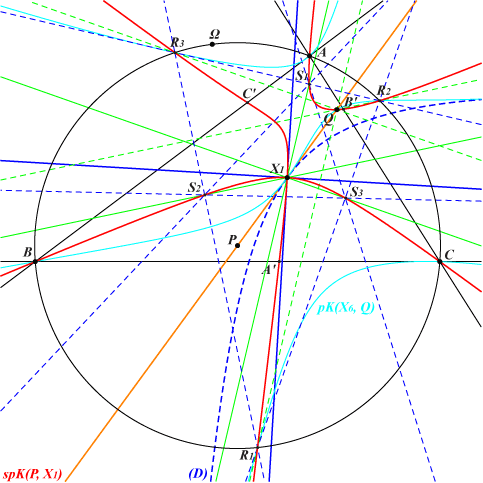Let P and Q be two fixed points such that Q is not on the line at infinity. Let L be a variable line through P and let L' be its reflection in Q. The isogonal transform L* of L intersects L' at two points M, N. When L varies, the locus of M, N is a circumcubic we shall call the sympivotal (isogonal, central) cubic spK(P, Q). Naturally, the isogonal conjugation can be replaced by any other isoconjugation and the results below are easily adapted. See CL045 with isotomic conjugation. See CL056 when the central symmetry is replaced by an axial symmetry and CL058 when it is replaced by a rotation. When we take Q = P (excluded in the sequel), the cubic is the usual isogonal pivotal cubic namely pK(X6, P) = spK(P, P). Notations : The prefixes c, a, t, g denote complement, anticomplement, isotomic, isogonal respectively.Equation and properties of spK(P, Q) With P = p : q : r and Q = u : v w, the equation of spK(P, Q) is : (u + v + w) ∑ [p x (b^2 z^2 - c^2 y^2)] - 2 (x + y + z) ∑ [a^2 (r v - q w) y z] = 0 or equivalently : ∑ [(2 u (q + r) - p (-u + v + w)) x (b^2 z^2 - c^2 y^2)] + 2 (a^2 y z + b^2 z x + c^2 x y) ∑ [(q w - r v) x] = 0. • spK(P, Q) contains the reflection P' of P about Q and the isogonal conjugate P* of P. The third point P3 of spK(P, Q) on the line P'P* lies on the circumconic that is the isogonal transform of the parallel at P to the line P'P*. P3 also lie on the line passing through P and the isogonal conjugate of the infinite point of the line P'P*, this latter point obviously on the circumcircle of ABC. The Simson line of this point is perpendicular to the line P'P*. • spK(P, Q) passe through A, B, C. The tangent at A is the isogonal transform of the line AA" where A" is the trace on BC of the parallel at P to the line AP'. • In general, spK(P, Q) does not contain P and Q. This is studied below. • The isogonal transform of spK(P, Q) is spK(P', Q). These two cubics generate a pencil that contains pK(X6, Q). It follows that these three cubics contain the four foci of the inconic I(Q) with center Q. With Q = X(39) see examples here, with Q = X(2) see Table 48, with Q = X(6) see Table 49, with Q = X(5) see Table 80. • spK(P, Q) meets the line at infinity at three points that also lie on pK(X6, P). These two cubics meet at six other points on the circumconic passing through P* and Q*, the isogonal transform of the line PQ. Apart A, B, C, these points are P* and two (not always real) isogonal conjugate points P1, P2 on the line PQ. These points consequently lie on pK(X6, Q). • spK(P, Q) meets the circumcircle at six points that also lie on pK(X6, P'). The two cubics meet at three other points namely P', P1, P2 as above. These six points are A, B, C and A', B', C' such that P' is the orthocenter of triangle A'B'C'. • Let La be the parallel at P' to the line AP. La meets BC at U and AP* at U'. These two points lie on the cubic spK(P, Q). The points V and V', W and W' are defined similarly. These properties are illustrated in the two figures below with P = X(5), Q = X(2) hence P' = X(549), P* = X(54), P1 = X(3), P2 = X(4).spK(P, Q) which are K0 cubics spK(P, Q) is a K0 (without term in x y z) if and only if the points K, P, Q are collinear. When P≠Q, this means that the line PQ contains K, the Lemoine point. In particular, spK(K,Q) and spK(P, K) are always K0 cubics. Note that spK(K,Q) contains G and the infinite points of the Grebe cubic K102 (see K527 for example) and spK(P, K) contains the foci of the inconic with center K. If Q≠K, we can take P at infinity on the line KQ. This gives an isogonal focal nK0 which is detailed below. The cubics spK(P on KQ, Q) form a pencil generated by pK(K, Q) = spK(Q, Q) and the focal cubic nK0 above. It follows that the pencil is globally invariant under isogonal conjugation. Moreover, two points P and P' symmetric about Q give two isogonal conjugate cubics spK(P, Q) and spK(P', Q). For example, with Q = G, we obtain the pencil generated by spK(G, G) = K002 (Thomson cubic) and spK(X524, G) = K018 (second Brocard cubic). This also contains spK(K, G) = K287 (a central cubic) and spK(X385, G) = K353. All these cubics contain G, K and the four foci of the Steiner inscribed ellipse.spK(P, Q) which are pivotal and/or pseudo-pivotal cubics spK(P, Q) is a pivotal cubic if and only if it is a K0 such that the triangles ABC and UVW are perspective. The triangle UVW is generally not a cevian triangle. This happens if and only if P lies on the central pK with center Q (see central cubics) in which case spK(P, Q) is a psK. In particular, this central pK contains Q, its anticomplement aQ and also aaQ, its pivot. It follows that spK(Q, Q) = pK(X6, Q) already mentioned, spK(aQ, Q) and spK(aaQ, Q) meet the sidelines of ABC at the vertices of a cevian triangle, that of Q, aQ and G respectively. Note that spK(aaQ, Q) is the isogonal transform of spK(aQ, Q) and recall that both cubics contain the foci of the inconic with center Q. These two cubics are pKs if and only if Q lies on the line GK. In this case, • spK(aQ, Q) = pK(gaQ, taQ) passes through G, H, K, gaQ, taQ, aaQ and is tangent at A, B, C to the symmedians, • spK(aaQ, Q) = pK(gtaQ, G) passes through G, O, K, aQ, gaaQ, gtaQ and the midpoints of ABC, • these two cubics belong to the pencil generated by K018 and pK(X6, Q) hence they also contain the four foci if the inconic I(Q) with center Q. The following table gives a selection of these cubics either by name or by a list of centers.Q spK(aQ, Q) = pK(gaQ, taQ) spK(aaQ, Q) = pK(gtaQ, G) X2 K002 K002 X6 K233 K168 X69 X2, X4, X6, X2996 X2, X3, X6, X193, X459, X3053 X81 X2, X4, X6, X1029 X2, X3, X6, X451, X1030, X2895 X86 X2, X4, X6, X2248 X2, X3, X6, X1654 X141 K141 K177 X230 K380 K357 X298 X2, X4, X6 X2, X3, X6, X3180 X299 X2, X4, X6 X2, X3, X6, X3181 X325 K354 K252 X333 X2, X4, X6 X2, X3, X6, X2305, X3144 X343 X2, X4, X6, X26, X68, X2165 X2, X3, X6, X24, X70, X571, X1993 X385 X2, X4, X6 X2, X3, X6, X420, X2076 X394 X2, X4, X6 X2, X3, X6, X1609 X395 K1145b K341b X396 K1145a K341a X524 K273 K043 X590 X2, X4, X6, X485, X3155 X2, X3, X6, X371, X492, X1585 X597 K283 K284 X599 X2, X4, X6 X2, X3, X6, X1384, X1992 X615 X2, X4, X6, X486, X3156 X2, X3, X6, X372, X491, X1586 X1211 X2, X4, X6, X37, X72, X321, X2895, X2915 X2, X3, X6, X28, X81, X1333 X1213 X2, X4, X6, X10, X42, X71, X199, X1654 X2, X3, X6, X27, X58, X86, X2248 X3068 X2, X4, X6, X1131 X2, X3, X6, X1151, X1270 X3069 X2, X4, X6, X1132 X2, X3, X6, X1152, X1271 X5743 K1135 K321 X6703 K379 K253 X11064 X2, X4, X6, X30, X323, X2986 K489Circular spK(P, Q) Since spK(P, Q) has the same points at infinity as pK(X6, P), it is a circular cubic if and only if P lies on the line at infinity. In this case, the cubic is an isogonal focal non-pivotal isocubic with singular focus F on the circumcircle, the isogonal conjugate of P. Its orthic line is the line PQ with trilinear pole Z and its root is ctZ. See Special Isocubics, §4.1.2. Recall that the cubic contains the foci of the inconic with center Q and, more generally, those of any inconic with center on PQ. See Table 74 when Q = X(5). When we take two parallel lines L, L', with same infinite point P, and symmetric about Q, their isogonal transforms L*, L'* are two circumconics passing through F. L* meets L' at M, N and L'* meets L at M*, N*. These four points lie on the cubic and the lines MN*, M*N pass through F. If L (hence L') contains Q, the points coincide two by two and lie on the axis (or orthic line) of the focal cubic.spK(P, Q) which are non-pivotal isocubics spK(P, Q) is a non-pivotal isocubic if the points U, V, W are collinear (necessary but usually not sufficient condition). These points U, V, W are collinear if and only if either P lies on the line at infinity – giving isogonal focal nK cubics, see above – or P lies on the circumconic with center Q. In this latter case, spK(P, Q) is a nK with root R on the circumconic with perspector Q. It is invariant in the isoconjugation swapping P' and P*. R is the second common point on this latter conic of the line passing through P' and the trilinear pole Z of the line Q, G/Q where G/Q is the center of the circumconic with perspector Q. The isoconjugate of R is the barycentric product of P* and aQ hence it lies on the trilinear polar of the isogonal conjugate of Q. The pole of spK(P, Q) is therefore also the barycentric product of R and its isoconjugate. This lies on cK(#X6, gaQ). For example, if we take Q = X(115), P lies on the Kiepert hyperbola then spK(P, Q) is a nK with pole on K222. See also K613.Equilateral spK(P, Q) Since spK(P, Q) has the same points at infinity as pK(X6,P), it is an equilateral cubic if and only if P = O and in this case, it has three real asymptotes parallel to those of the McCay cubic and concurring at X such that HX = 2/3 HQ. Furthermore, this cubic must contain H. spK(O, Q) is therefore a stelloid and also contains the reflection O' of O about Q and meets the McCay cubic at two other points P1, P2 on the line OQ and on the rectangular circum-hyperbola that is its isogonal transform. This cubic is actually the locus of point M such that O', M and the isogonal conjugate of M with respect to the pedal triangle of M are collinear. Note that when Q = O, spK(O, Q) is the McCay cubic itself. When Q lies on the Brocard axis OK, spK(O, Q) is a K0 and belongs to the pencil generated by the McCay cubic and the union of the line at infinity and the Kiepert hyperbola. See Table 51. spK(O, Q) is a K60++ if and only if Q lies on the homothetic of K026 under h(H,3/2). In particular, this gives the cubics spK(O, H) = K525, spK(O, X140) = K026 and spK(O, X550) = K080. spK(O, Q) meets the sidelines of ABC at the vertices of a cevian triangle if and only if Q lies on the homothetic of K044 under h(O, 1/2). This contains the points X(3), X(5), X(140), X(389), X(1216) giving the cubics K003, K028, K026, K049, K071.Circumnormal spK(P, Q) Since spK(P, Q) has the same points on the circumcircle as pK(X6,P'), it is a circumnormal cubic if and only if P' = O i.e. if and only if Q is the midpoint of OP. In such case, the cubic contains O, the vertices of the circumnormal triangle (these four points on the McCay cubic) hence there are two remaining common points on these two cubics. They are the intersections P1, P2 of the line OP with the rectangular circumhyperbola passing through P*. These two points are isogonal conjugates but they are not necessarily real. When P = O, spK(P, Q) is the McCay cubic itself. Note that any circumnormal spK is actually the isogonal transform of an equilateral spK and conversely. Here is a selection of such circumnormal cubics : K003, K009, K361, K373, K405. See also Table 25.spK(P, Q) passing through P spK(P, Q) contains P if and only if Q lies on the line at infinity (giving circular cubics as already said) or on the line PP*. This gives two situations : • if P is an in/excenter, the line PP* is undefined hence any cubic spK(X1, Q) contains X1 and similarly when P is an excenter. spK(X1, Q) has three asymptotes parallel to the (internal) bisectors of ABC. • if P is not an in/excenter, the cubics spK(P, Q on PP*) form a pencil which can be generated by pK(K, P) and the decomposed cubic into the line at infinity and the circumconic passing through P and P*. All these cubics have the same infinite points and generally the same tangent at P*, that to the conic. When Q is the midpoint of PP*, spK(P, Q) is a nodal cubic with node P* and spK(P*, Q) is a nodal cubic with node P, each being the isogonal transform of the other. P must not lie on the circumcircle or on the line at infinity in order to get a proper undecomposed cubic. For example spK(X3, X5) = K028 (third Musselman cubic) and spK(X4, X5) = K009 (Lemoine cubic). When Q = P*, spK(P, Q) = spK(P, P*) is a central cubic with center P*. See K526 for example. Equivalently, for given P distinct of an in/excenter not lying on the circumcircle or on the line at infinity, spK(P*, midpoint of PP*) is a nodal cubic with node P and spK(P*, P) is a central cubic with center P. Recall that both cubics have the same points at infinity, namely those of pK(X6, P*). The table gives a selection of such cubics.P* P nodal cubic central cubic pK(X6, P*) X(2) X(6) K281 K314 K002 X(3) X(4) K028 K525 K003 X(4) X(3) K009 K443 K006 X(6) X(2) K280 K287 K102 X(54) X(5) X(5), X(54) K526 pK(X6, X54) X(55) X(7) X(7), X(55), X(991), X(1000), X(2550) X(7), X(55) pK(X6, X55) X(58) X(10) X(10), X(58), X(65), X(1201) X(10), X(58) pK(X6, X58) X(69) X(25) X(25), X(69), X(206), X(376) X(25), X(69) K169 X(385) X(694) X(385), X(511), X(694), X(3228) X(385), X(511), X(694) K128The nodal cubics spK(P, X1) spK(P, X1) is a nodal cubic with node X(1), the nodal tangents being perpendicular. These are parallel to the asymptotes of the diagonal rectangular hyperbola (D) passing through the in/excenters and tangent at X(1) to the line X(1)P. Its center Ω is the isogonal conjugate of the infinite point of the trilinear polar of the barycentric quotient X1 ÷ P. Note that spK(X1, X1) decomposes into the internal bisectors of ABC hence we consider P ≠ X(1) in the sequel.If Q denotes the reflection of P in X(1), then spK(P, X1) meets (O) at A, B, C and three points R1, R2, R3 that lie on pK(X6, Q). Recall that Q is the orthocenter of R1R2R3. spK(P, X1) meets the sidelines of R1R2R3 again at S1, S2, S3 which are the orthogonal projections of X(1) on these sidelines. spK(P, X1) meets the sidelines of ABC again at A', B', C'. A' lies on the parallel at Q to the line AP and B', C' are defined likewise. *** Remarkable nodal tangents They are parallel to the asymptotes of the Jerabek, Kiepert, Feuerbach hyperbolas if and only if P lies on the trilinear polar of X(662), X(799), X(190) respectively. These trilinear polars pass through X(1) since the three points lie on the circum-conic with peerspector X(1).Special cases • when P lies on the line at infinity, spK(P, X1) is an isogonal strophoid of CL003. • when P lies on the circum-conic with center X(1), spK(P, X1) is also a nK but it splits into a line and a circum-conic both passing through X(1) and meeting again on the cubic K407 = cK(#X1, X57). • when P lies on the line X(1)X(6), spK(P, X1) is a K0. • when P lies on the cubic K201 = pK(X9, X145), spK(P, X1) is a psK with pseudo-pivot on K365 = pK(X57, X7) and pseudo-isopivot on K761 = pK(X41, X1) which is the isogonal transform of K365. Naturally, X(1) can be replaced with an excenter and the properties above are easily adapted under extraversion. The following table gives a selection of remarkable cubics spK(P, X1) for some finite points P. See CL003 when P is at infinity.P type cubic / centers remarks nodal tangents parallel to the asymptotes of X(2) -- X(1), X(6), X(7), X(999), X(3241) Feuerbach hyperbola X(3) -- K594 a stelloid X(6) K0 X(1), X(2), X(3242) X(8) psK K360 = psK(X56, X7, X1) a Lemoine cubic Feuerbach hyperbola X(9) K0 X(1), X(57), X(3243) X(10) -- K915 Feuerbach hyperbola X(11) -- X(1), X(7), X(56), X(59), X(1317) X(21) X(1), X(65), X(79), X(1432) Jerabek hyperbola X(63) X(1), X(19), X(2093), X(3062) Jerabek hyperbola X(75) X(1), X(31), X(33), X(2263) Kiepert hyperbola X(78) X(1), X(34), X(84), X(1420) Feuerbach hyperbola X(145) psK K259 = psK(X55, X2, X1) Feuerbach hyperbola X(200) X(1), X(269), X(1743), X(3062) Feuerbach hyperbola X(519) K086 an isogonal strophoid Feuerbach hyperbola X(758) X(1), X(484), X(758), X(759), X(3065) Jerabek hyperbola X(956) K0 X(1), X(4), X(957) X(1001) K0 X(1), X(7), X(1002) X(1043) X(1), X(225), X(1042), X(3214) Kiepert hyperbola X(1317) -- X(1), X(8), X(11), X(55), X(1318) X(1482) -- X(1), X(3) a CircumNormal cubic X(2398) X(1), X(885), X(2401), X(2424) Feuerbach hyperbola X(3241) X(1), X(2), X(55), X(1000) Feuerbach hyperbolaThe isogonal transform of spK(P, X1) is spK(P', X1) where P' is the reflection of P about X(1). These two cubics are represented by cells of the same color.Other special cases of cubics spK(P, Q) spK(cQ, Q) or spK(P, aP) : see K755 and CL066.